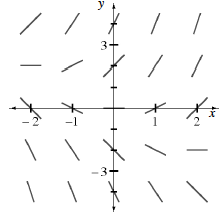### Home > CALC > Chapter 9 > Lesson 9.2.2 > Problem9-68

9-68.

Examine the slope field for $\frac { d y } { d x } = x + y$  for $−2 ≤ x ≤ 2$ below.1. If $y(−2) = 2$, use Euler's Method to draw a solution curve for $y$ using $∆x = 1$.

2. Draw a new solution using Euler's Method if $y(−2) = 0$.

Start at $(–2, 2)$. $dy/dx = 0$, so the next point is $(–1, 2)$.
Using $(–1, 2)$, $dy/dx = 1$, so the next point is $(0, 3)$.
Continue this process to locate more points on the solution curve.